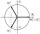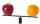# Im>0?

Is -10i a positive number?

Result

#### Solution:

No, complex (imaginary) numbers are not ordered and therefore does not make sense to compare them.

Leave us a comment of example and its solution (i.e. if it is still somewhat unclear...):

Showing 0 comments:Be the first to comment!#### To solve this example are needed these knowledge from mathematics:

Try our complex numbers calculator.

## Next similar examples:

1. Imaginary numbersFind two imaginary numbers whose sum is a real number. How are the two imaginary numbers related? What is its sum?
2. Complex number coordinatesWhich coordinates show the location of -2+3i
3. Linear imaginary equationGiven that ? "this is z star" Find the value of the complex number z.
4. ABS CNCalculate the absolute value of complex number -15-29i.
5. ReciprocalCalculate reciprocal of z=0.8-1.8i:
6. De Moivre's formulaThere are two distinct complex numbers z such that z3 is equal to 1 and z is not equal 1. Calculate the sum of these two numbers.
7. Median and modusRadka made 50 throws with a dice. The table saw fit individual dice's wall frequency: Wall Number: 1 2 3 4 5 6 frequency: 8 7 5 11 6 13 Calculate the modus and median of the wall numbers that Radka fell.
8. Is complexAre these numbers 2i, 4i, 2i + 1, 8i, 2i + 3, 4 + 7i, 8i, 8i + 4, 5i, 6i, 3i complex?
9. Theorem proveWe want to prove the sentense: If the natural number n is divisible by six, then n is divisible by three. From what assumption we started?
10. CompareCompare with characters >, <, =: 85.57 ? 80.83
11. Sequence 3Write the first 5 members of an arithmetic sequence: a4=-35, a11=-105.
12. DiscriminantDetermine the discriminant of the equation: ?
13. RootsDetermine the quadratic equation absolute coefficient q, that the equation has a real double root and the root x calculate: ?
14. 75th percentile (quartille Q3)Find 75th percentile for 30,42,42,46,46,46,50,50,54
15. Six termsFind the first six terms of the sequence a1 = -3, an = 2 * an-1
16. Linsys2Solve two equations with two unknowns: 400x+120y=147.2 350x+200y=144
17. EquationEquation ? has one root x1 = 8. Determine the coefficient b and the second root x2.